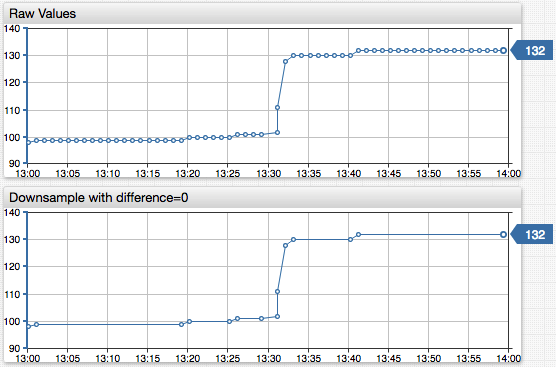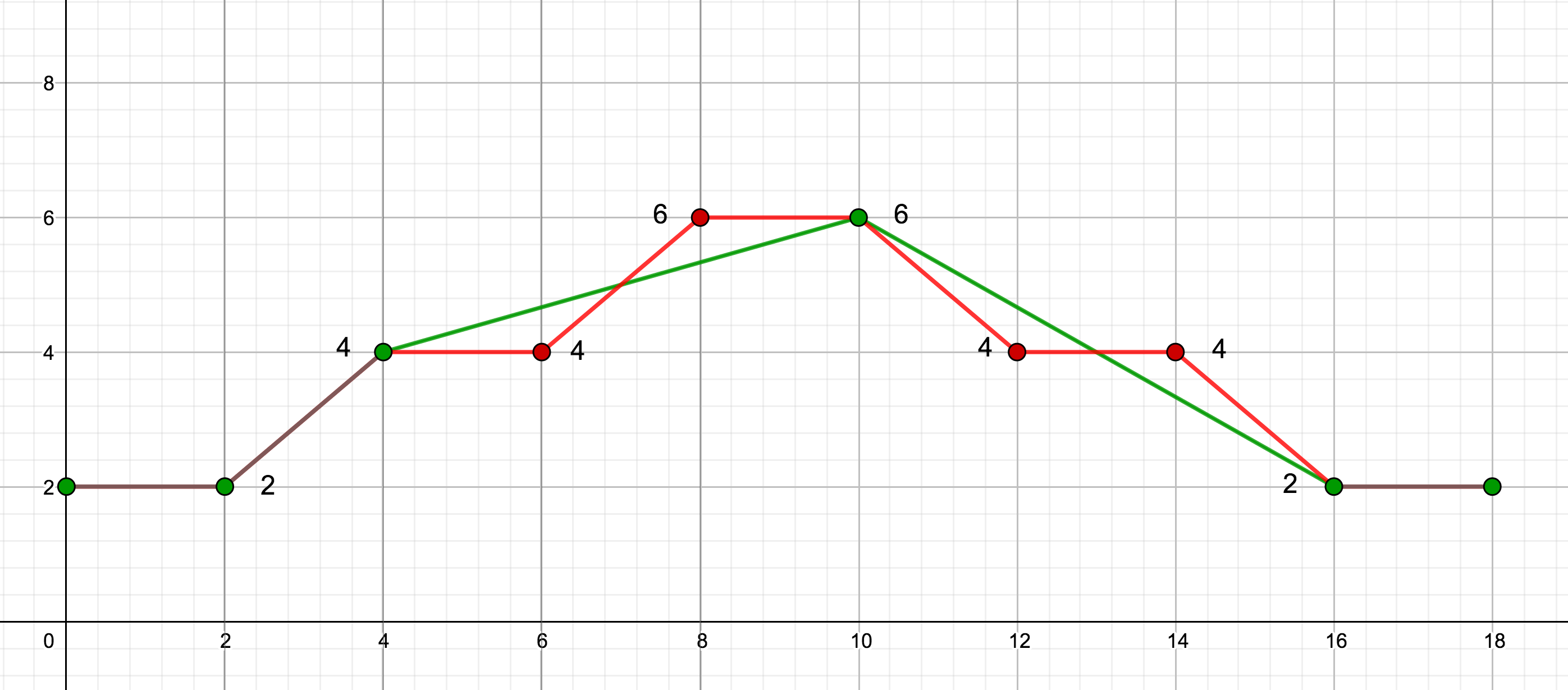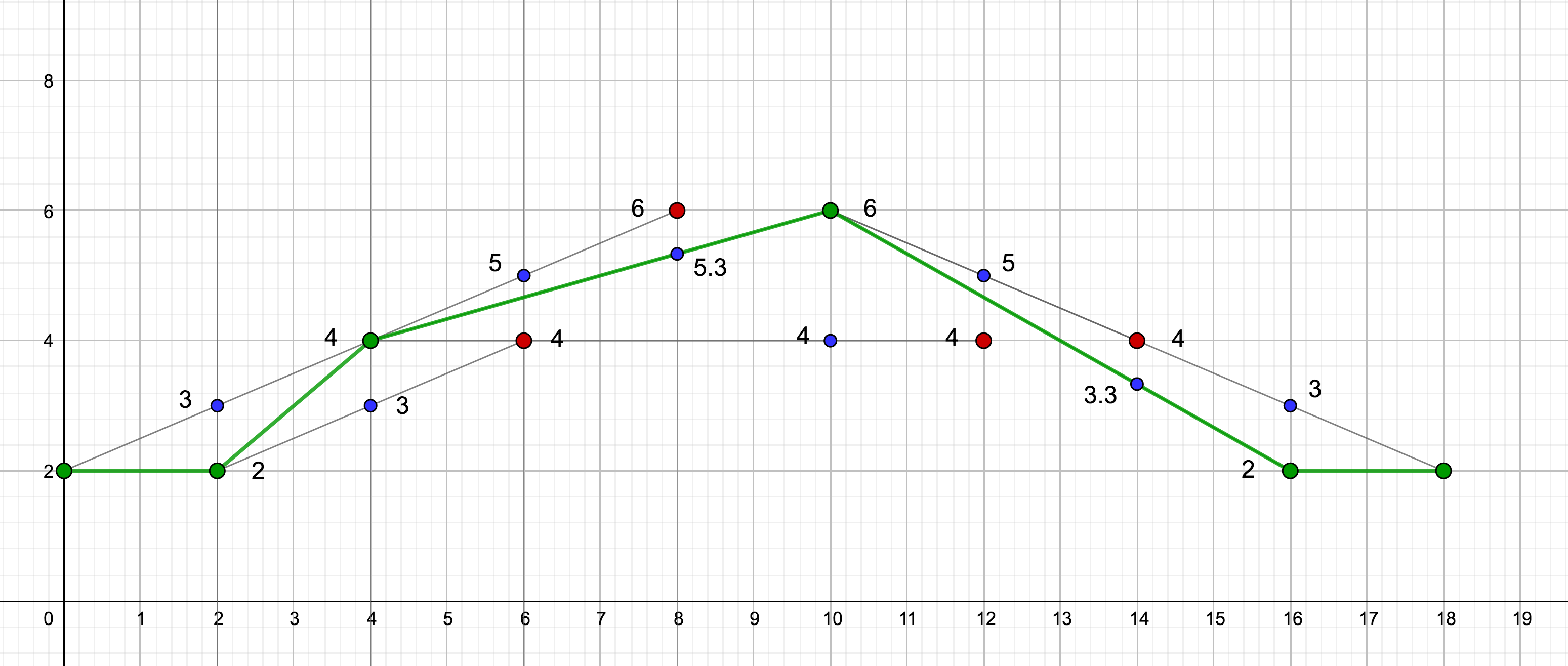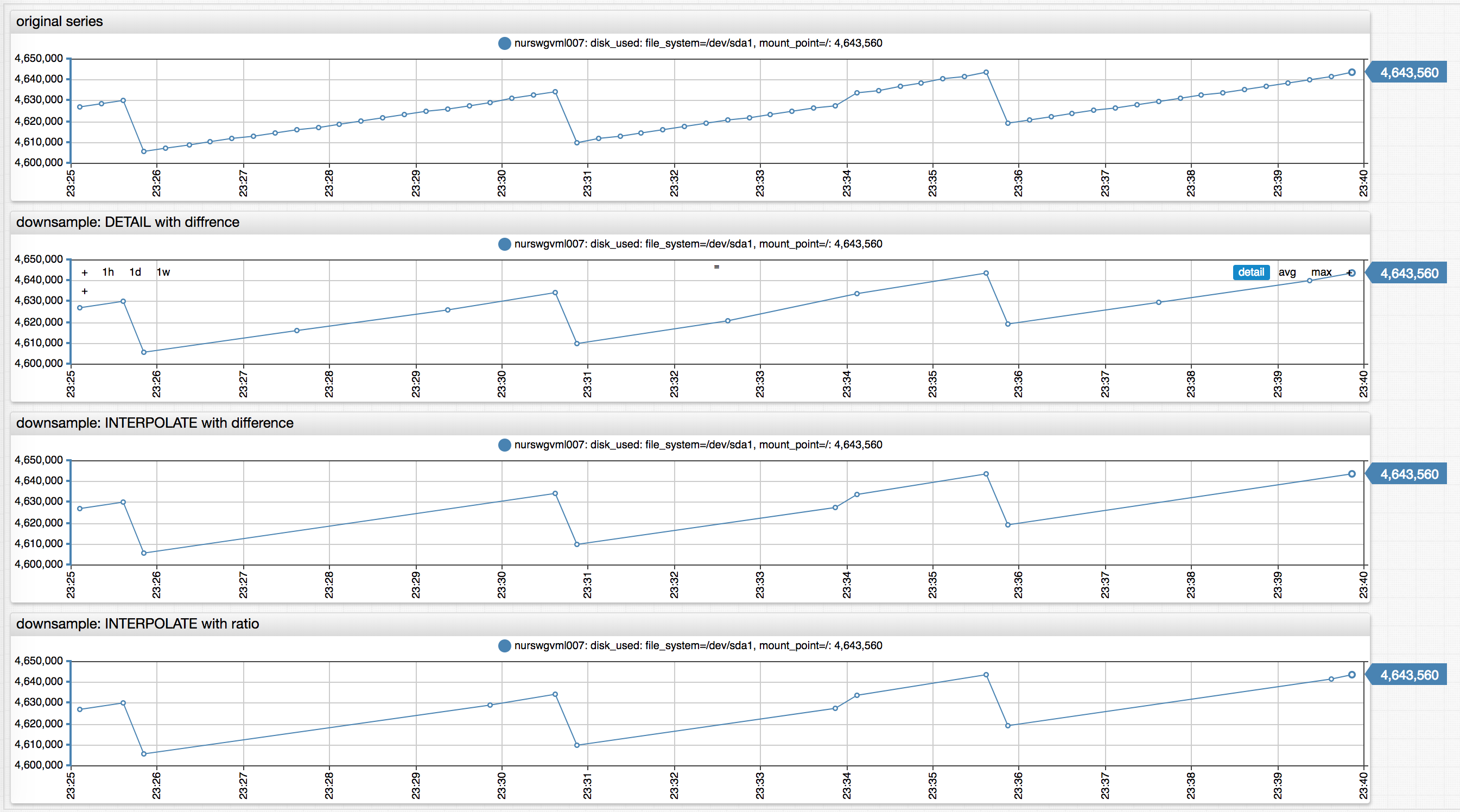# # Downsampling

## # Overview

Downsampling transformation reduces time series density by filtering out sequentially duplicate samples from a response.

### # Basic Example

``````"downsample": {
"difference": 0
}
``````

The configuration example excludes samples that are equal to the previous and next values.``````"downsample": {
"algorithm": "INTERPOLATE",
"difference": 10
}
``````

The configuration excludes samples that are within `±10` of an interpolated value.

## # Parameters

Name Type Description
`algorithm` string Downsampling algorithm determines which values to discard as duplicate.
Possible values: `DETAIL` or `INTERPOLATE`.
Default: `DETAIL`.
`difference` number The sample is classified as duplicate if the current value deviates by more than the specified difference, in absolute terms, from the estimated value produced by downsampling algorithm.
Minimum value: `0`.
Default: `0`.
`ratio` number The sample is classified as duplicate if the ratio of the current value and the value produced by downsampling algorithm or the inverse exceed the specified ratio.
Minimum value: `1`.
Default: `none`.
`gap` object Maximum distance between subsequent samples in the transformed series. Specified as count and time unit.
Default: `none`.

TIP

The `difference` and `ratio` parameters cannot be specified simultaneously.

## # Gap

If the `gap` parameter is specified, and the time distance between the current and last returned sample exceeds the gap, the current sample is always included in the result.

Name Type Description
`count` number Number of time units.
`unit` string Time unit, for example `MINUTE`.
``````"gap": {"count": 2, "unit": "MINUTE"}
``````

The `gap` parameter prevents a time series from becoming too sparse as a result of downsampling.

## # Processing

The samples in the input series are evaluated sequentially in ascending time order.

The following samples are always included in the result, even if classified as duplicate by the downsampling algorithm.

• The first and the last samples.
• Annotated samples: Sample with a non-empty text field.
• Samples with `NaN` value.
• Samples whose previous or next sample value is `NaN`.

The remaining samples are checked by the algorithm which excludes them from the results if classified as duplicates and the provided `gap` condition is satisfied.

If the query retrieves versioned series, the algorithm evaluates the latest version. If the latest version is classified as a duplicate, then all versions with the same timestamp are classified as such.

## # Algorithm

The `DETAIL` and `INTERPOLATE` algorithms use different formulas to classify samples as duplicates.

This documentation references the following keywords and definitions:

• `sample`: Current evaluated sample.
• `last_sample`: Last returned series sample included in the result.
• `next_sample`: Sample following current `sample`.

The timestamps of these samples are `time`, `last_time`, `next_time`, and their values are `value`, `last_value`, `next_value`.

In addition, the `INTERPOLATE` algorithm performs linear interpolation between the `last_sample` and the `next_sample` to calculate `interpolated_value` with a timestamp equal to `time`.

### # Ratio Check

When the `ratio` parameter is set, the algorithms calculate several multiples.

• `DETAIL` algorithm:

• `value/last_value`
• `last_value/value`
• `value/next_value`
• `next_value/value`
• `INTERPOLATE` algorithm:

• `value/interpolated_value`
• `interpolated_value/value`

The sample is classified as a duplicate if all multiples do not exceed the specified `ratio`.

Note

To avoid division by zero, the algorithm compares `x/ratio > y` instead of `x/y > ratio`.

### # Difference Check

If the `ratio` parameter is not set, the algorithm uses absolute difference to classify the sample. If the difference `parameter` is not set, it defaults to `0`. The algorithm classifies the sample as duplicate if the following expressions return `true`.

• `DETAIL` algorithm:
``````abs(value - last_value) <= difference AND abs(value - next_value) <= difference
``````

If the difference is `0`, the sample is a duplicate if it is equal both to the last and the next value:

``````value == last_value AND value == next_value
``````
• `INTERPOLATE` algorithm:
``````abs(value - interpolated_value) <= difference
``````

## # Examples

### # Default Downsampling

``````"downsample": {}
``````

Result:

``````|       | input  | downsampled |
| time  | series |   series    |
|-------|--------|-------------|
| 07:00 |   1    |      1      | first sample
| 08:00 |   1    |      -      |
| 09:00 |   1    |      -      |
| 10:00 |   1    |      -      |
| 11:00 |   1    |      -      |
| 12:00 |   1    |      1      | differs from next sample
| 13:00 |   2    |      2      | differs from last returned sample
| 14:00 |   2    |      -      |
| 15:00 |   2    |      2      | differs from next sample
| 16:00 |   3    |      3      | differs from last returned sample
| 17:00 |   3    |      -      |
| 18:00 |   3    |      -      |
| 19:00 |   3    |      -      |
| 20:00 |   3    |      3      | last sample
``````

### #`DETAIL` downsampling with `difference` and `gap`

``````"downsample": {
"difference": 1.5,
"gap": {"count": 4, "unit": "HOUR"}
}
``````

Result:

``````|       | input  | downsampled |
| time  | series |   series    |
|-------|--------|-------------|
| 07:00 |   1    |      1      | first sample
| 08:00 |   1    |      -      |
| 09:00 |   1    |      -      |
| 10:00 |   1    |      -      |
| 11:00 |   1    |      -      |
| 12:00 |   1    |      1      | time gap with last returned sample exceeds 4 hours
| 13:00 |   2    |      -      |
| 14:00 |   2    |      -      |
| 15:00 |   2    |      -      |
| 16:00 |   3    |      3      | differs from last returned sample (1.0 at 12:00) by 2.0
| 17:00 |   3    |      -      |
| 18:00 |   3    |      -      |
| 19:00 |   3    |      -      |
| 20:00 |   3    |      3      | last sample
``````

### #`INTERPOLATE` downsampling

``````"downsample": {
"algorithm": "INTERPOLATE"
}
``````

Result:

``````|       | input  | downsampled |
| time  | series |   series    |
|-------|--------|-------------|
| 07:00 |   1    |      1      | first sample
| 08:00 |   3    |      -      |
| 09:00 |   5    |      -      |
| 10:00 |   7    |      -      |
| 11:00 |   9    |      9      | last sample
``````

### #`INTERPOLATE` downsampling with `ratio`

``````"downsample": {
"algorithm": "INTERPOLATE",
"ratio": 1.25
}
``````

Result:

``````|       | input  | downsampled | interpolated |       |
| time  | series |   series    |   value      | ratio |
|-------|--------|-------------|----------------------|
|   00  |   2    |      2      |      -       |   -   | first sample
|   02  |   2    |      2      |      3       | 1.50  | ratio exceeds threshold
|   04  |   4    |      4      |      3       | 1.30  | ratio exceeds threshold
|   06  |   4    |      -      |      5       | 1.25  |
|   08  |   6    |      -      |     5.3      | 1.13  |
|   10  |   6    |      6      |      4       | 1.50  | ratio exceeds threshold
|   12  |   4    |      -      |      5       | 1.25  |
|   14  |   4    |      -      |     3.3      | 1.21  |
|   16  |   2    |      2      |      3       | 1.50  | ratio exceeds threshold
|   18  |   2    |      2      |      -       |   -   | last sample
``````

Charts of the input and downsampled series are provided below. The input series is colored red, the downsampled series is colored green.The chart illustrates how the `INTERPOLATE` algorithm calculates interpolated values. Interpolated values are colored blue.ChartLab Examples